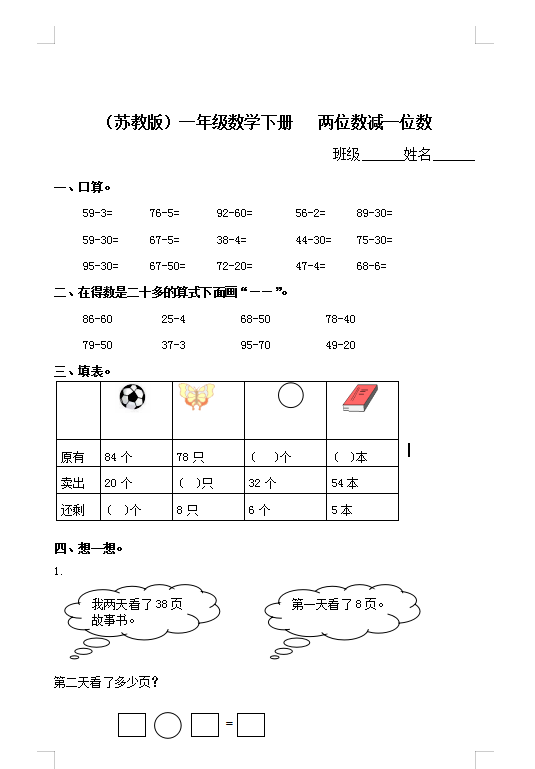（苏教版）小学一年级数学下册 两位数减一位数及答案 1（4页DOC文档）资料下载

（苏教版）小学一年级数学下册   两位数减一位数

59-3=      76-5=      92-60=       56-2=     89-30=

59-30=     67-5=      38-4=        44-30=    75-30=

95-30=     67-50=     72-20=       47-4=     68-6=